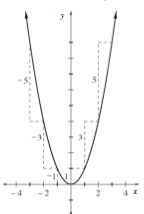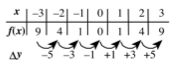### Home > CALC > Chapter 2 > Lesson 2.2.2 > Problem2-68

2-68.

If you plotted the finite differences of a parabola, the result would be what type of function?

Review problem 1-96.

Recall that $Δy$ represents the finite difference. What pattern do you see among the finite differences of the parabola $y = x^²$? What would the graph of those finite difference look like? Is this true for all parabolas?